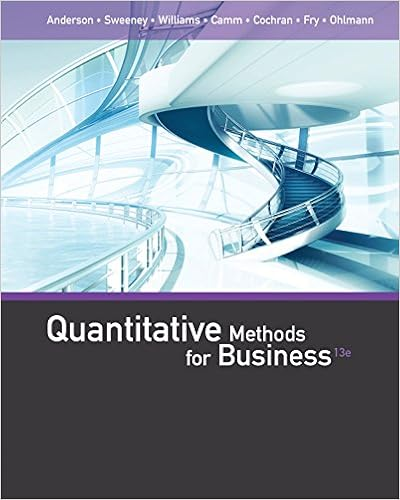# A stylist at the hair care palace gathered data on

• 4
• 50% (2) 1 out of 2 people found this document helpful

This preview shows page 3 - 4 out of 4 pages.

##### We have textbook solutions for you!
The document you are viewing contains questions related to this textbook.The document you are viewing contains questions related to this textbook.
Chapter 3 / Exercise 15
Anderson/SweeneyExpert Verified
14. A stylist at the Hair Care Palace gathered data on the number of hair colorings givenon Saturdays and weekdays.Her results are listed below.Assume the two sampleswere taken independently:Saturdays:n1= 50x1= 14weekdays:n2= 65x2= 13a.Find the Point estimate ofP1-P2and the margin of error.b.Estimate the difference in the true proportions with a 99% confidence interval.Interpret this interval.15. IfXhas an exponential distribution with parameterλ, derive a general expression forthe (100p)th percentile of the distribution. Then specialize to obtain the median.16. Find the joint and marginal densities corresponding to the cdfF(x, y) = (1-e-αx)(1-e-βy)x0y0α >0β >0.3
##### We have textbook solutions for you!
The document you are viewing contains questions related to this textbook.The document you are viewing contains questions related to this textbook.
Chapter 3 / Exercise 15Expert Verified
•••